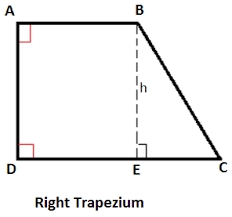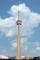# Calculate 7

Calculate the height of the trapezoid ABCD, where coordinates of vertices are:
A[2, 1], B[8, 5], C[5, 5] and D[2, 3]

h =  1.6641

### Step-by-step explanation:Did you find an error or inaccuracy? Feel free to write us. Thank you!Tips to related online calculators
Line slope calculator is helpful for basic calculations in analytic geometry. The coordinates of two points in the plane calculate slope, normal and parametric line equation(s), slope, directional angle, direction vector, the length of the segment, intersections of the coordinate axes, etc.
Pythagorean theorem is the base for the right triangle calculator.

#### You need to know the following knowledge to solve this word math problem:

We encourage you to watch this tutorial video on this math problem:

## Related math problems and questions:

• Coordinates of square verticesI have coordinates of square vertices A / -3; 1/and B/1; 4 /. Find coordinates of vertices C and D, C and D. Thanks, Peter.
• SquarePoints A[9,9] and B[-4,1] are adjacent vertices of the square ABCD. Calculate the area of the square ABCD.
• Coordinates of square verticesThe ABCD square has the center S [−3, −2] and the vertex A [1, −3]. Find the coordinates of the other vertices of the square.
• Find parametersFind parameters of the circle in the plane - coordinates of center and radius: ?
• IS trapezoidCalculate the length of diagonal u and height v of isosceles trapezoid ABCD, whose bases have lengths a = |AB| = 37 cm, c = |CD| = 29 cm and legs b = d = |BC| = |AD| = 28 cm.
• Square 2Points D[10,-8] and B[4,5] are opposed vertices of the square ABCD. Calculate area of the square ABCD.
• Rectangular trapezoidIn a rectangular trapezoid ABCD with right angles at vertices A and D with sides a = 12cm, b = 13cm, c = 7cm. Find the angles beta and gamma and height v.
• Isosceles triangleIn an isosceles triangle ABC with base AB; A [3,4]; B [1,6] and the vertex C lies on the line 5x - 6y - 16 = 0. Calculate the coordinates of vertex C.
• EllipseEllipse is expressed by equation 9x2 + 25y2 - 54x - 100y - 44 = 0. Find the length of primary and secondary axes, eccentricity, and coordinates of the center of the ellipse.
• Rectangular trapezoidThe rectangular trapezoid ABCD is: /AB/ = /BC/ = /AC/. The length of the median is 6 cm. Calculate the circumference and area of a trapezoid.
• Points on circleIn the Cartesian coordinate system with the origin O is a sketched circle k /O; r=2 cm/. Write all the points that lie on a circle k and whose coordinates are integers. Write all the points that lie on the circle I / O; r=5 cm / and whose coordinates are
• Circle and squareAn ABCD square with a side length of 100 mm is given. Calculate the radius of the circle that passes through the vertices B, C and the center of the side AD.
• The sides 2The sides of a trapezoid are in the ratio 2:5:8:5. The trapezoid's area is 245. Find the height and the perimeter of the trapezoid.
• Rectangular trapezoidThe ABCD rectangular trapezoid with the AB and CD bases is divided by the diagonal AC into two equilateral rectangular triangles. The length of the diagonal AC is 62cm. Calculate trapezium area in cm square and calculate how many differs perimeters of the
• TrianglePlane coordinates of vertices: K[11, -10] L[10, 12] M[1, 3] give Triangle KLM. Calculate its area and its interior angles.
• A cell towerA cell tower is located at coordinates (-5, -7) and has a circular range of 12 units. If Mr. XYZ is located at coordinates (4,5), will he be able to get a signal?
• Find the 13Find the equation of the circle inscribed in the rhombus ABCD where A[1, -2], B[8, -3] and C[9, 4].